# Compound Stoichiometry formula composition formula Percent Composition copper

• Slides: 18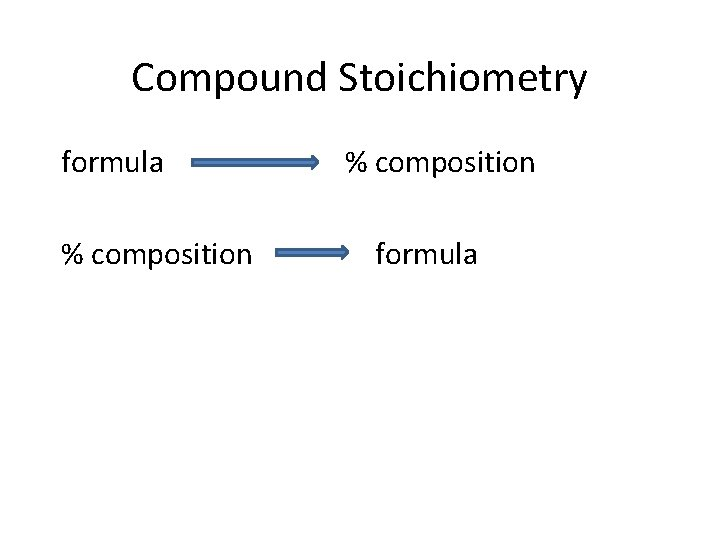Compound Stoichiometry formula % composition formula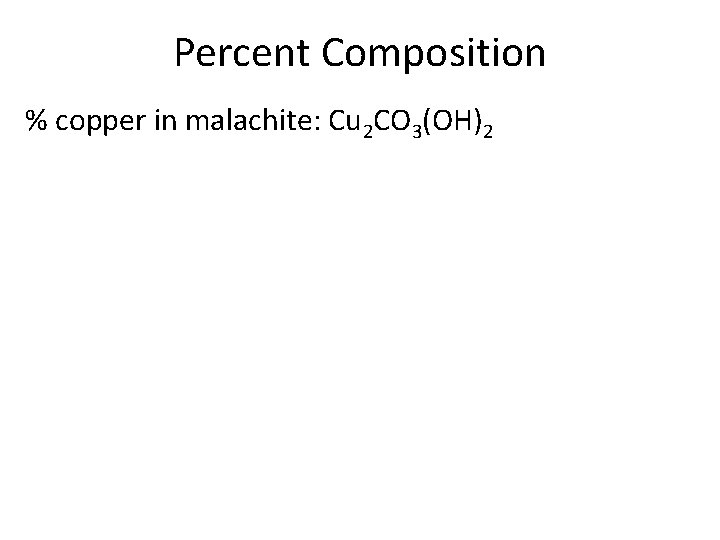Percent Composition % copper in malachite: Cu 2 CO 3(OH)2Percent Composition % copper in malachite: Cu 2 CO 3(OH)2Using Percent Composition A compound contains: 43. 6% P 56. 4% S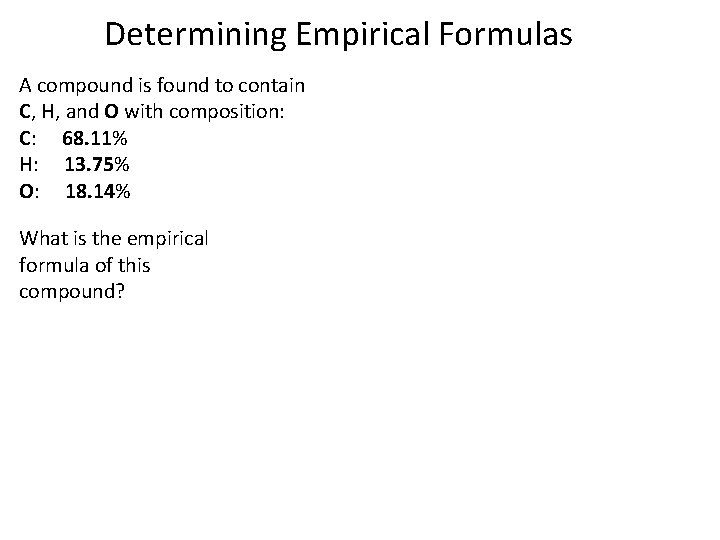Determining Empirical Formulas A compound is found to contain C, H, and O with composition: C: 68. 11% H: 13. 75% O: 18. 14% What is the empirical formula of this compound?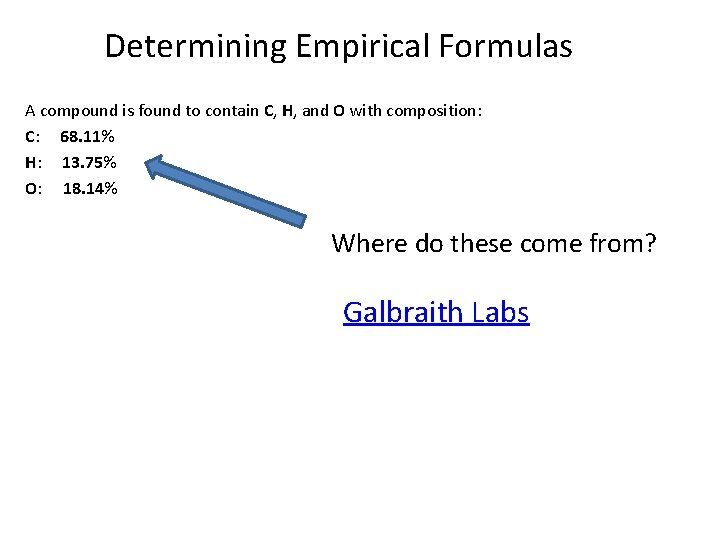Determining Empirical Formulas A compound is found to contain C, H, and O with composition: C: 68. 11% H: 13. 75% O: 18. 14% Where do these come from? Galbraith Labs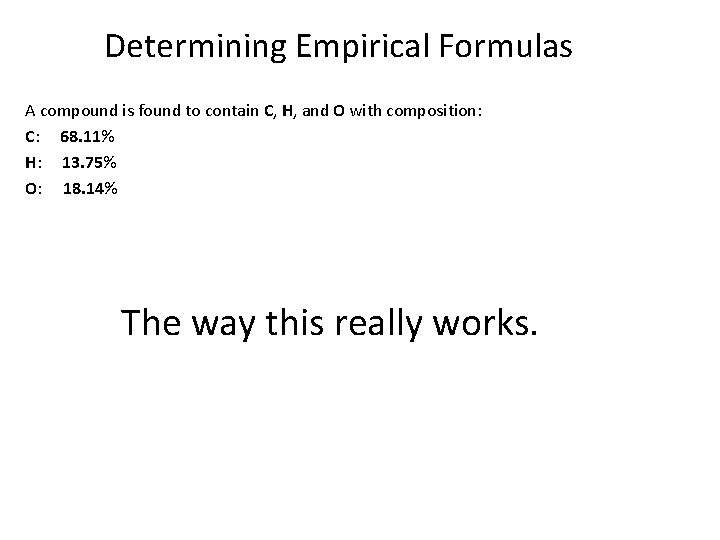Determining Empirical Formulas A compound is found to contain C, H, and O with composition: C: 68. 11% H: 13. 75% O: 18. 14% The way this really works.Determining Empirical Formulas The way this really works. ic 302222 qSolving Hard Problems 4. 56 g of a compound containing Fe and P is reacted so that the P is converted to phosphate ion, PO 43 -. It is then precipitated by forming an ionic compound with Ca 2+ ions. The mass of the calcium phosphate has a mass of 6. 16 g. What is the empirical formula of the original compound?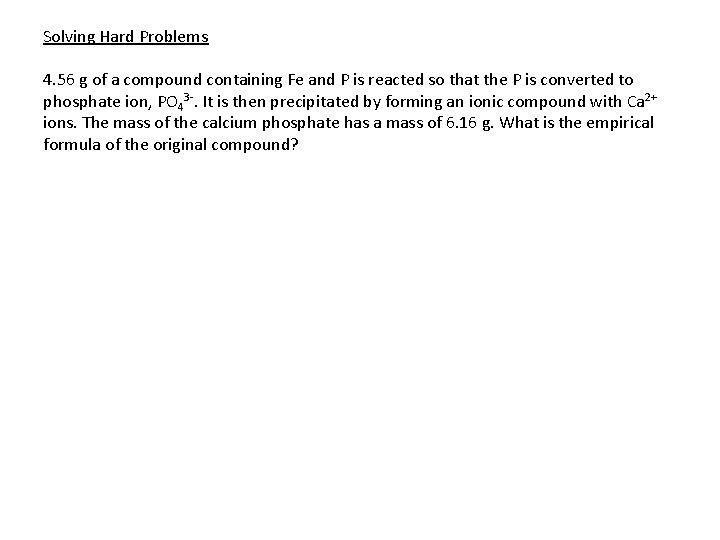Solving Hard Problems 4. 56 g of a compound containing Fe and P is reacted so that the P is converted to phosphate ion, PO 43 -. It is then precipitated by forming an ionic compound with Ca 2+ ions. The mass of the calcium phosphate has a mass of 6. 16 g. What is the empirical formula of the original compound?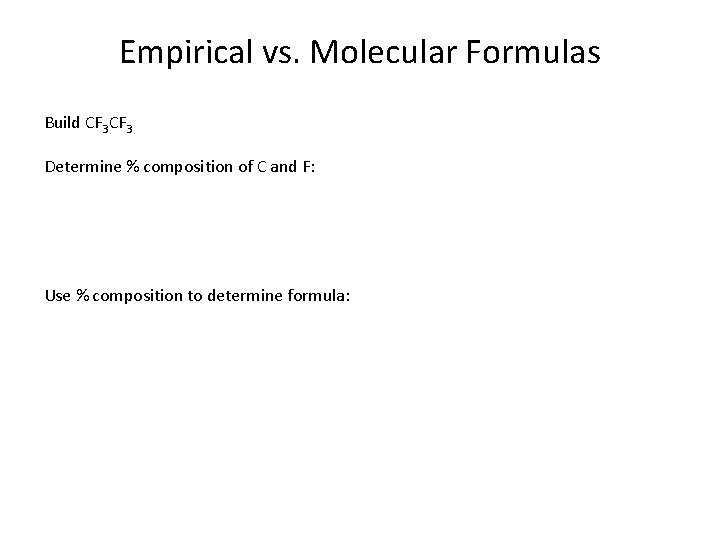Empirical vs. Molecular Formulas Build CF 3 Determine % composition of C and F: Use % composition to determine formula:Empirical vs. Molecular FormulasDetermining Molecular Formulas A compound is: P: 15. 12% N: 6. 841% Br: 78. 04% Molar mass = 614. 3 g/mol What is the molecular formula? Part 1. Determine empirical formula to be PNBr 2.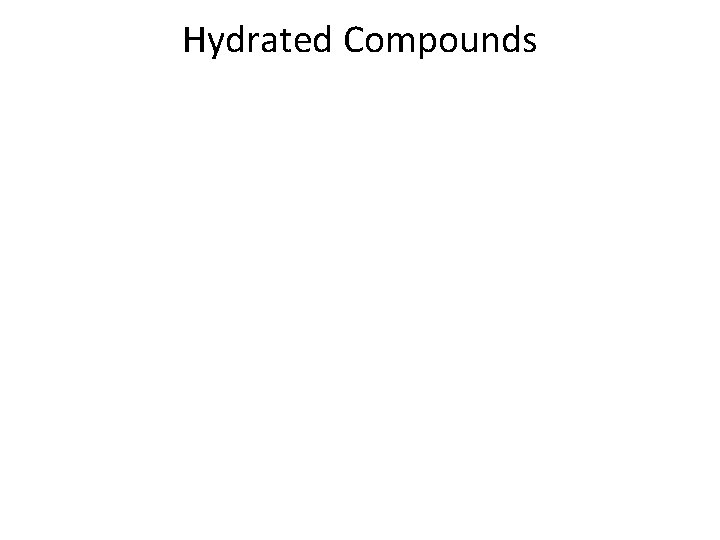Hydrated CompoundsHydrated Compounds A compound is: Co. Cl 2 x H 2 O Mass before heating: 32. 86 g Mass after heating: 17. 93 g What is x?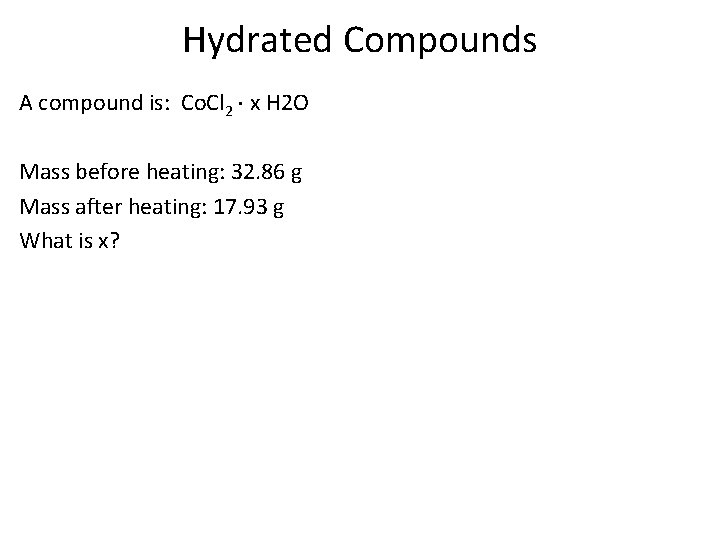Hydrated Compounds A compound is: Co. Cl 2 x H 2 O Mass before heating: 32. 86 g Mass after heating: 17. 93 g What is x?